# Texas Go Math Grade 3 Lesson 13.4 Answer Key Divide by 9

Refer to our Texas Go Math Grade 3 Answer Key Pdf to score good marks in the exams. Test yourself by practicing the problems from Texas Go Math Grade 3 Lesson 13.4 Answer Key Divide by 9.

## Texas Go Math Grade 3 Lesson 13.4 Answer Key Divide by 9

Essential Question
What strategies can you use to divide by 9?

Unlock the Problem
Becket’s class goes to the aquarium. The 27 students from the class are separated into 9 equal groups. How many students are in each group?

• Do you need to find the number of equal groups or the number in each group?

One Way
Make equal groups.

• Draw 9 circles to show 9 groups.
• Draw 1 counter in each group.
• Continue drawing 1 counter at a time until all 27 counters are drawn.There are __ counters in each group.
So, there are ___ in each group.
You can write 27 ÷ 9 = ___ or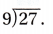Math Talk
Mathematical Processes
What is another way you could solve the problem? Explain.

Other Ways
A. Use factors to find 27 ÷ 9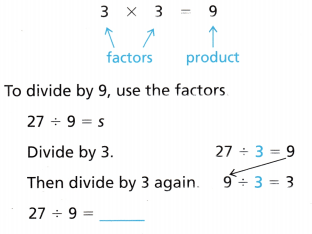B. Use a related multiplication fact.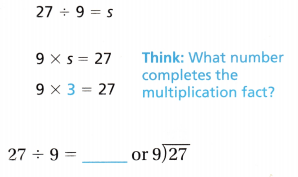Math Talk
Mathematical Processes
Explain how you would use factors to find 18 ÷ 9.

Share and Show

Question 1.
Draw counters in the groups to find 18 ÷ 9. ___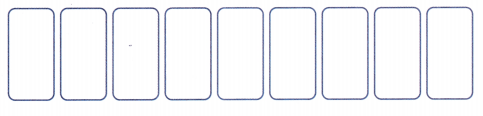Find the quotient.

Question 2.
__ = 45 ÷ 9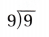Question 4.Question 5.
__ = 54 ÷ 9

H.O.T. Algebra Complete the table.

Question 6.Question 7.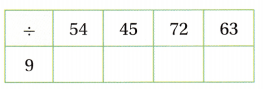Unlock the Problem

Question 8.
H.O.T. Multi-Step Carlos has 28 blue tang fish and 17 yellow tang fish in one large fish tank. He wants to separate the fish so that there are the same number of fish in each of 9 smaller tanks. How many tang fish will Carlos put in each smaller tank?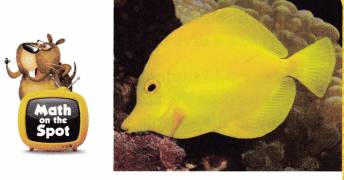a. What do you need to find?

b. Evaluate Why do you need to use two operations to solve the problem?

c. Write the steps to find how many tang fish Carlos will put in each smaller tank.

d. Complete the sentences.
Carlos has ___ blue tang fish and yellow tang fish in one large fish tank.
He wants to separate the fish so that there are the same number of fish in each of __ smaller tanks.
So, Carlos will put __ fish in each smaller tank.

Question 9.
Multi-Step Sophie has two new fish. She feeds one fish 4 pellets and the other fish 5 pellets each day. If Sophie has fed her fish 72 pellets, for how many days has she had her fish? Explain.

Fill in the bubble for the correct answer choice.

Question 10.
There are 45 students going on a field trip. For every 9 students on the field trip, there will be 1 adult. How many adults are going on the field trip?
(A) 5
(B) 9
(C) 1
(D) 10

Question 11.
Baseball games have 9 innings. The Little Tigers played 72 innings last season. How many games did the Little Tigers play last year?
(A) 9
(B) 6
(C) 8
(D) 5

Go Math Lesson 13.4 Divide by 9 Answer Key Question 12.
Multi-Step There are a total of 72 panes in the large windows and a total of 44 panes in the small windows at the city museum. Each large window has 9 panes. Each small window has 4 panes How many windows are there at the city museum?
(A) 8
(B) 19
(C) 3
(D) 11

Texas Test Prep

Question 13.
At the aquarium, Ms. Brady separates 36 students into 9 equal groups. How many students are in each group?
(A) 2
(B) 5
(C) 4
(D) 3

### Texas Go Math Grade 3 Lesson 13.4 Homework and Practice Answer Key

Find the unknown number.

Question 1.
___ = 72 ÷ 9

Question 2.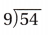Question 3.
___ = 27 ÷ 9

Question 4.
___ ÷ 9 = 2

Question 5.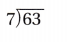Question 6.
9 ÷ ___ = 1

Complete the table.

Question 7.Question 8.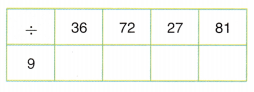Problem Solving

Question 9.
Daryl collects 38 plastic liter bottles and 43 plastic jugs. He divides them equally into 9 recycling bins. How many plastic containers does he put into each bin? Write the steps to solve the problem.

Question 10.
Annette has 27 pink buttons, 36 red buttons, and 45 green buttons. How can she sort the buttons into 9 equal piles, so there are the same number of different color buttons in each pile? Explain.

Texas Test Prep

Lesson Check

Question 11.
Jamal has 63 marbles. For every 8 blue marbles, there is 1 red marble. How many red marbles does Jamal have?
(A) 5
(B) 6
(C) 9
(D) 7

Question 12.
Anquan made 45 party favors. He puts an equal number of favors on 9 tables. How many party favors are on each table?
(A) 6
(B) 5
(C) 7
(D) 8

Go Math Grade 3 Practice and Homework Lesson 13.4 Answer Key Question 13.
Carla has a coin collection of 54 coins. She puts 9 coins into each page of a coin book. How many pages does Carla fill in her coin book?
(A) 9
(B) 7
(C) 6
(D) 8

Question 14.
There are 9 people at the diner. Their bill totals $72. They split the bill into equal amounts. How much money does each person spend? (A)$8
(B) $9 (C)$6
(D) \$7

Question 15.
Multi-Step Suzie has 36 daffodils, 27 tulips, and 18 roses. She wants to make 9 bouquets with an equal number of flowers. How many flowers will Suzie put into each bouquet?
(A) 9
(B) 2
(C) 3
(D) 4